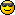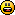# Math Is Fun Forum

Discussion about math, puzzles, games and fun.   Useful symbols: ÷ × ½ √ ∞ ≠ ≤ ≥ ≈ ⇒ ± ∈ Δ θ ∴ ∑ ∫  π  -¹ ² ³ °

You are not logged in.

## #1 Re: Puzzles and Games » Add 13 more and post it forever. » 2010-04-12 09:32:44

2691= 2^4 + 3^4 + 3^4 + 5^4 + 5^4 + 5^4 + 5^4 + 13## #2 Re: Help Me ! » Complex Analysis » 2009-12-29 10:17:04

the +b is just a translation, the hard part is the az. Try polar coordinates.

## #3 Re: Puzzles and Games » Geometry problem, nice solution » 2009-08-19 08:00:33

JaneFairfax wrote:

Vi har

och
. Om
.
.

Det är ett skönt problem. Tack så mycket, Kurre.Yes that is the trigonometric approachGood job!

Have you been studying swedish? I think I remember that you wrote that you are interested in languages.Nicely done!

Anyway here is my method:

Reflect AB in the horizontal line and draw line BC as in 2. Now reflect the triangle ABC in AC to make a quadrilateral as in 3. Now, all angles in the quadrilateral ABCD are equal, and all sides are equal, thus it is a square, and since AC is a diagonal,

must be half of a right angle, ie

## #4 Re: Puzzles and Games » Geometry problem, nice solution » 2009-08-19 06:06:51

1a2b3c2212 wrote:

HOW TO READ THAT LANGUAGE??? can translate into english pls? thx

I did translate the important parts of the text:

Kurre wrote:

Find

Altough I should maybe have written that the figure consists of three squares, but that is kind of obvious.

## #5 Re: Puzzles and Games » Root between (0,1)? » 2009-08-18 08:27:25

TheDude wrote:

Right?

No. You forget that there is a minus sign. We have 3c<-b. But:

there is a minus sign infront of the 3c/2, so we cant apply the inequality there.

## #6 Puzzles and Games » Geometry problem, nice solution » 2009-08-17 09:14:35

Kurre
Replies: 4

http://g.imagehost.org/0615/3462.jpg
Find

This is an easy problem if you use the standard approach with trigonometry (the problem is for ~17 years old students). But I found a much nicer geometrical method, without using any trigonometry.
So my challenge for you is to find it.(or solve it any other way without trigonometry is fine too)

## #7 Re: Help Me ! » Sum of n-th degrees » 2009-08-14 21:40:17

Look at mathsyperson proof of the sum of squares:
http://www.mathisfunforum.com/viewtopic.php?pid=18619#p18619
This proof can be generalized so, if you know the formula for all sums with powers up to k, we can express the sum to the power k+1 in the other sums.

## #8 Re: Puzzles and Games » Root between (0,1)? » 2009-08-13 10:17:44

Im not following. You cant just substitute expressions for inequalities in other inequalities

TheDude wrote:

\\

Substitute these values into our previous inequality:

The Right hand side is ok, but not the left side. You have:

if im not missing anything.

## #9 Re: Help Me ! » nice fact » 2009-08-11 03:33:10

Il give you some tips. First prove that the first number (4-digit minus reverse) is divisible by 9. We know (or you can prove that too) that a number is divisible by 9 iff its sum of digits is divisible by 9. So when we first add the digits it must be a number divisible by 9. What possible numbers are there?

## #10 Re: Help Me ! » prove it! » 2009-08-10 12:25:21

We first use the cosine theorem on each of the squared sides:

Thus we get:

solving for
and using the areaforumla (
etc) :

To minimize this sum we can for example use jensens inequality.
http://en.wikipedia.org/wiki/Jensen%27s_inequality
We first assume that the triangle is acute, so all angles are less than
. Then
is convex, since
and
. Thus Jensens inequality applies and we get:

so

What if one angle is obtuse?
Then we can argue as follows. WLOG we assume

. Then there exists a corresponding triangle with sides a',b',c' and
and b=b' and c=c'. This triangle must have the same area since
but a'<a since it corresponds to a smaller angle. the new triangle is acute, so we can apply the result there, thus

Q.e.d

## #11 Re: Help Me ! » Find p: » 2009-06-23 06:11:56

use the formula on http://en.wikipedia.org/wiki/Trig_identities#Linear_combinations

## #12 Re: Help Me ! » There are infinitely many primes p such that p + 2 is also prime. » 2009-06-22 04:23:48

Ricky wrote:

I guess he means that, because we assumed that p,p+2 where the largest pair there does not exist any numbers with factors p+2k,p+2(k+1).

As I posted before, this statement is false.  (p+2k)*(p+2(k+1)) has precisely those numbers as factors.

hm I meant prime factors, but maybe he does not use that they are prime factors, idk, im too lazy to read through the oproof atm.

## #13 Re: This is Cool » seven and thirteen » 2009-06-17 00:22:18

There are two different accounts posting here, both RICKisanidiot and RICKYisanidiot, imo a clear violence to the multiple account rule (which I think exist on this forum?)

## #14 Re: Help Me ! » There are infinitely many primes p such that p + 2 is also prime. » 2009-06-16 22:16:25

Ricky wrote:

I'm having a lot of trouble understanding what it is you're trying to go for.

If we suppose that there exist a larger pair of the form p+2k and p+2(k+1) Than the set of numbers that have as factors p+2k and  p+2(k+1) must be equal to 0 (since they do not exist).

We are supposing that there exists a larger pair where p+2k and p+2(k+1) are both prime?  This doesn't make sense, we've already supposed that p and p+2 were the largest pair with this property.

And you say that the number of integers that have p+2k and p+2(k+1) as factors must be zero.  This is false, just look at the number:

(p+2k)*(p+2(k+1))

This number has both those as a factor.

I guess he means that, because we assumed that p,p+2 where the largest pair there does not exist any numbers with factors p+2k,p+2(k+1). IM not sure though and I have not tried to understand the proof either.

## #15 Re: Exercises » trigonometri problem » 2009-06-10 04:15:29

I wanted to find a solution that uses the result from problem 3, which took  a while and is probably not the easiest one, but here it is anyway:

## #17 Re: Help Me ! » Geometry drawing environment » 2009-05-31 22:10:48

Thanks for the tips!
Actually I made this thread to see what kinds of programs that existed, since I got the idea to create such a program myself. I have tried Geometers Sketchpad and Cabri now, and Cabri was really nice but was not exactly the type of program I had in mind, altough I guess I will not be able to make a program close as good. But Il maybe give it a try anyway## #18 Help Me ! » Geometry drawing environment » 2009-05-28 23:56:03

Kurre
Replies: 6

Are there any good programs for drawing geometrical pictures? I mean that has functions for lets say creating circumcircles, marking centroids/circumcentres/medians etc, basically a program that is designed for creating proofs/problems/solutions in euclidean geometry??

## #20 Re: Exercises » Kurre's Exercises » 2009-05-24 21:40:00

#15let k,n be positive integers, a a nonzero real, k<n+1 . Show that:

both with real analysis and by using residue calculus

edit: i did a mistake so i dont know if its possible to do this using residues, but that does not mean it must be impossible

## #21 Re: Help Me ! » Prove, using comittee-forming... » 2009-05-24 18:33:52

what do you mean by block walking?## #22 Re: Help Me ! » Prove, using comittee-forming... » 2009-05-24 11:13:48

Write it as C(n,0)*C(n,n)+C(n,1)*C(n,n-1)+...+C(n,k)*C(n,n-k)+...+C(n,n)*C(n,0).
Then assume you have a set of 2n persons, and divide it into two sets A and B of n persons in each. Now you want to choose a comittee of n persons. Then for each choice there will be say k persons in set A, and n-k persons in set B, and summing will yield exactly the sum above.

## #23 Re: Help Me ! » Triangular prisms » 2009-05-24 11:06:48

eh how does one define length, width and height for a triangular prism?? :s

## #24 Re: Exercises » Kurre's Exercises » 2009-05-23 00:31:08

kean wrote:

sure！ it's right

\sum_{k=1}^n \zeta_k^m =0

it does not hold for all m and n